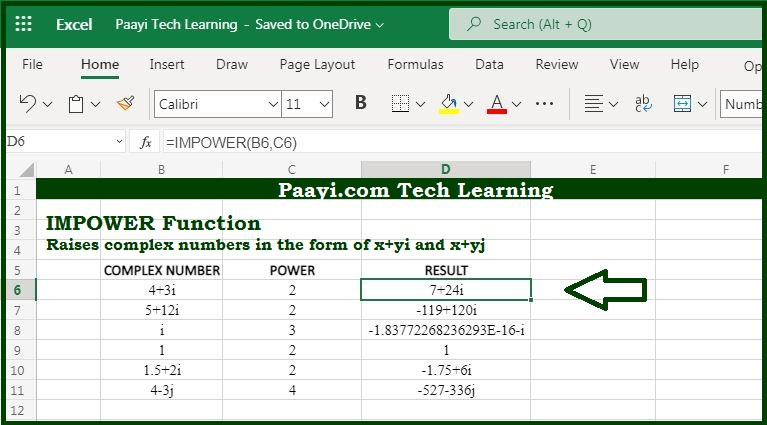# Learn How to Use Microsoft Excel IMPOWER Function

Written by | 0 Comments | 676 Views

In this article, you will learn how to use the Microsoft Excel IMPOWER function and its prime function in Microsoft Excel. You will also get to know the Microsoft Excel IMPOWER function return value and syntax with the help of some examples.

Microsoft Excel IMPOWER Function

The prime function of Microsoft Excel IMPOWER function is to raise the complex number to a given power. So, with the help of IMPOWER function you can able to return the raised complex number to a given power. The complex number should be in the form of  "x + yi" or "x + yj". You can also use the COMPLEX function to create complex numbers from real and imaginary parts.

Return Value of IMPOWER Function

The return value will be the complex number as a text.

Syntax of IMPOWER Function

=IMPOWER(inumber, number)

Where the arguments:

inumber: It is the complex number.

number: It is the power to raise the number.

## How to Use Microsoft Excel IMPOWER Function?As we know the IMPOWER function you can able to return the raised complex number to a given power. The complex number is input in the form text as,  "x + yi" or "x + yj". It should be noted that only lowercase "i" and "j" are accepted by the IMPOWER function and other values will result in a #NUM! error. The number arguments should always be numeric.